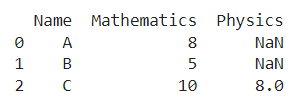# How To Count The NaN Values In A Column In Pandas DataFrame

A DataFrame is the primary data structure of the Pandas library in Python and is commonly used for storing and working with tabular data. A common operation that could be performed on such data is to count the NaN values in a Pandas DataFrame in order to extract more information from it.

To start working with Pandas, we first need to import it:

``import pandas as pd``

We’ll also need the NumPy library for this to specify NaN values. To work with NumPy, we first need to import it in the following manner:

``import numpy as np``

## Running Example

Let us understand this operation with the help of an example. Consider the following DataFrame containing 3 students with names A, B, and C and their corresponding marks (out of 10) for two subjects, Mathematics and Physics.Code snippet for generating the above DataFrame:

``````import pandas as pd
import numpy as np

# Dictionary for our data
data = {'Name' : ['A', 'B', 'C'], 'Mathematics' : [8, 5, 10], 'Physics' : [np.nan, np.nan, 8]}

# DataFrame for the dictionary
df = pd.DataFrame.from_dict(data=data)

# Printing the DataFrame
print(df)``````

Here, data is a dictionary we created to initialize the DataFrame. For this, we use the DataFrame() function of the Pandas library which takes the dictionary as an argument and returns the required DataFrame. One important thing to note here is that the first two rows of the column physics have NaN values.

Now, let’s say we need to count the number of NaN values we have in the physics column in order to maybe gather some more information about the dataframe at hand.

The resulting output would look like this:

2

Let us look at different ways of performing this operation on a given DataFrame:

## How To Count The NaN Values In Pandas Column

In this method, we use the value_counts() function to count the number of nan values in a column of interest here physics.

First, we use df[‘Physics’] to access the physics column. Then we apply the value_counts() function to it and then access the count of nan values using np.nan enclosed within square brackets.

Let us take a look at the corresponding code snippet and generated output for this method:

``````# Importing required libraries
import pandas as pd
import numpy as np

# Dictionary for our data
data = {'Name' : ['A', 'B', 'C'], 'Mathematics' : [8, 5, 10], 'Physics' : [np.nan, np.nan, 8]}

# DataFrame for the dictionary
df = pd.DataFrame.from_dict(data=data)

# Performing the operation
cnt = df['Physics'].value_counts(dropna = False)[np.nan]

# Printing the result
print(cnt)``````

Output :

2

Instead of using df[‘Physics’] to access the physics column, we can also use df.Physics to access it and still get the same results.

Let us take a look at the corresponding code snippet and generated output for this method:

``````# Importing required libraries
import pandas as pd
import numpy as np

# Dictionary for our data
data = {'Name' : ['A', 'B', 'C'], 'Mathematics' : [8, 5, 10], 'Physics' : [np.nan, np.nan, 8]}

# DataFrame for the dictionary
df = pd.DataFrame.from_dict(data=data)

# Performing the operation
cnt = df.Physics.value_counts(dropna = False)[np.nan]

# Printing the result
print(cnt)``````

Output :

2

## 2. Count The NaN Values Using the .isnull() function in Pandas

In this method, we use the isnull() function to count the number of nan values in a column of interest, here physics.

First, we use df[‘Physics’] to access the physics column. We apply the isnull() function to this which gives True or False values appropriately, i.e. True if nan and False if not nan.

Then we apply the value_counts() function to it and then access the count of True values using True enclosed within square brackets.

Let us take a look at the corresponding code snippet and generated output for this method :

``````# Importing required libraries
import pandas as pd
import numpy as np

# Dictionary for our data
data = {'Name' : ['A', 'B', 'C'], 'Mathematics' : [8, 5, 10], 'Physics' : [np.nan, np.nan, 8]}

# DataFrame for the dictionary
df = pd.DataFrame.from_dict(data=data)

# Performing the operation
cnt = df['Physics'].isnull().value_counts()[True]

# Printing the result
print(cnt)``````

Output :

2

Instead of using square brackets to access the column values, we can also use simple dot operators. For example, row.Physics in place of row[‘Physics’]. Let us take a look at the python code and the corresponding output for this method:

``````# Importing required libraries
import pandas as pd
import numpy as np

# Dictionary for our data
data = {'Name' : ['A', 'B', 'C'], 'Mathematics' : [8, 5, 10], 'Physics' : [np.nan, np.nan, 8]}

# DataFrame for the dictionary
df = pd.DataFrame.from_dict(data=data)

# Performing the operation
cnt = df.Physics.isnull().value_counts()[True]

# Printing the result
print(cnt)``````

Output :

2

## Conclusion

In this topic, we have learned to count the number of NaN values in a column of an existing Pandas DataFrame, following a running example of test scores of students in different subjects, thus giving us an intuition of how this concept could be applied in real-world situations. Feel free to reach out to info.javaexercise@gmail.com in case of any suggestions.

Trending